# Frequency-Severity Modeling in consideration of COVID-19 induced effects

This post is supposed to give a brief introduction in Frequency-Severity models. These models are very popular for determine the optimal price for an insurance. We will take a look at the general idea of frequency-severity modeling and for illustration explore a simple example by using rudimentary assumptions. There is a vast amount of literature that deals with how to calculate the model putting different assumptions. For example by assuming that frequency and severity is not independent . To model covariates this article uses Generalized Linear Models (GLM)  and as an extension Generalized Linear Mixed Models (GLMM), which could be used to model corona effects, solved using Maximum likelihood estimation. Other methods use Bayesian methods , with the advantage that asymptotic results can be derived even if the observed data is sparse, or tree methods that allows a non linear relationship [4, 5].

## 1. Frequency-Severity Modeling

For illustration purposes we lay out a simple univariate model (we are going to model only a single risk), extending the model is straightforward. We want to model the Loss Cost of a given entity. Letbe the number of claims over the time interval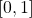,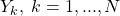, the amount of each claim (loss). Our aim is model the aggregated loss, sinceis unbounded, for asymptotics, it is meaningful to consider the average loss per claim. By construction we are facing two random variables,is continues whileis discrete, the idea of Frequency-Severity Modeling is to model the joined distributionas product of frequency and conditional severity distributionInstead one can also modeldirectly, often done using a Tweedie distribution, however by separating frequency and severity additional insights are expected.

### 1.1 Example

A popular approach is to modelas a compound Poisson process. Assume thatis a counting of a Poisson process with intensity, whilewhere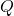is a probability distribution with density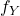independent of. Thenand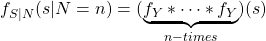a popular choice here is to assume a gamma distribution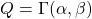which leads tofor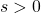andif. Statistical measures of interest are for example expected loss and variance given by

(1)#### 1.1.1 Estimation

Assume we observed claims, then the maximum likelihood estimators are given by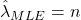,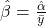, while an optimalhas to fulfillwhere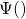is Digamma function . In practice this equation is solved numerically. This leads to estimators for expectation and variance.

## 2. Introducing Covariates

The previous analysis was carried out without covariates. In the insurance context, there is usually more information available than just the amount and number of claims. Covariates are for example the age of a customer or the car type when looking at motor insurance contracts. An important aspect when modeling insurance data is how long a contract had existed in a certain state of covariates. Thus additionally we introduce an artificial covariates labeled as “exposure” used to calibrate the size of a potential outcome variable. Based on these covariates we are interested in the conditional expectation.

### 2.1 Modeling covariates using GLM

A common way to model covariates is to use a GLM. Let the outcomebe a random variable with a particular distribution of the exponential family, e.g. the density can be written aswhereis a scaling parameter also known as dispersion parameter. We assume that there exists some link functionsuch that for outcomewith independent covariates,. Besides, a nice feature is that the variance function has the form

(2)meaning that the variance is a function of the mean.

For estimation we rely on Maximum likelihood, in general the log likelihood for the-th covariate givenobservations given by

(3)using the identities,.

#### 1.2.1 Estimate the example using GLM

Recap our previous example, by using a compound Poisson process it was assumed thatis a counting of a Poisson process whilefollows adistribution. It is easy to verify, that both distributions are member of the exponential family since the distribution of the Poisson process is given by,,while for the Gamma distribution we have to use,,. Finally, based on (1) and (2),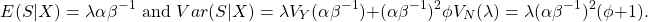We assume that we observetypes of policyholder. Since in this example, the observed claimsper policyholder is independent from the corresponding claim amountthe two GLM are estimated separately. Further we assume that there existscovariates. We use a log-link for either GLM, such that withthe link function is given byand thus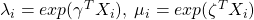whereand.

In order to find the maximum likelihood estimate for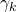, we use the log likelihood to calculate

(4)with a limiting distribution.
Accordingly the maximum likelihood estimate for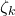using the log likelihood is given by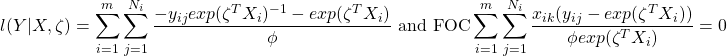with a limiting distribution.

### 2.2 Incorporate Random Effects

Mixed effect models are used in cases where the response variables arise from different distributions. Therefore they are particularly useful provided that a repeated measurement is made on the same or on clusters of related statistical units. A nice intuition whether to model a random or a fixed effect can be found here. In a nutshell, model a factor as a random effects if:

• If the factor (group) is expected to have expression not yet observed (not entire population of groups is observed)
• If for a particular reason we are not interested in the coefficient of the factor rather than the anticipated structure that may be imposed on our observations.

In the insurance context we can use random effects to model a setup where several observations belong to the same few policyholder. In that case the unique identifier for each policyholder is used as covariates for random effects. Another important application where we may use random effect to create better models is due to the corona crisis. Here we observe polices which where issued under corona restrictions installed by the government while others are not. It is very likely that, for example because people do no longer drive to work, this has an effect on the occurred claims. A possible way out is to add the information of government policies as additional covariates to be modeled using random effects.
Thus, as an extension of the GLM in this section we will now include both fixed and random effects, leading to a Generalized Mixed Effect Model (GLMM). Similar to the GLM case we assume that each expression offollows a particular distribution of the exponential family such that conditioned on the random effect(5)where the covariates are separated for fixed effects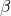and random effects, distributed with, such that,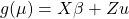. For random effects we require that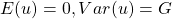. Similar to (3) the likelihood is given by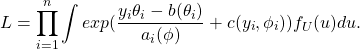Therefore fitting GLMMs via maximum likelihood is very similar to solve the GLM, but involves an additional integration over the random effects. This is usually extremely computationally intensive, especially when more covariates are modeled using random effects.

#### 2.2.1 Extension of the Example

For illustration we will now extend our frequency example using a Poisson process and allow further for a single independent normal distributed random effect. Thus

(6)Using the link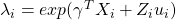, the Likelihood is comparable to (4) and is given by## 3. Coding example in R

As an illustrative example we will use the well know Swedish Insurance Dataset. These data were compiled by the Swedish Committee on the Analysis of Risk Premium in Motor Insurance, summarized in Hallin and Ingenbleek (1983) and Andrews and Herzberg (1985). The data are cross-sectional, describing third party automobile insurance claims for the year 1977. In this example we will model the number of claims (the frequency) using a GLM as described above based on the covariates Kilometers, Zone, Bonus, Make and with a GLMM using “Zones” as random effects.

library('lme4')
## Loading required package: Matrix
library('doParallel')
## Loading required package: foreach
## Loading required package: iterators
## Loading required package: parallel
library('foreach')

# Fit the Model using the entire Dataset to check for significant factors
glm =glm(Claims ~ Kilometres+factor(Zone)+
summary(glm)
##
## Call:
## glm(formula = Claims ~ Kilometres + factor(Zone) + factor(Bonus) +
##     factor(Make), family = poisson(link = log), data = SwedishMotorInsurance,
##     offset = log(Insured))
##
## Deviance Residuals:
##     Min       1Q   Median       3Q      Max
## -7.9809  -0.8745  -0.1800   0.5774   6.5738
##
## Coefficients:
##                 Estimate Std. Error  z value Pr(>|z|)
## (Intercept)    -1.915417   0.014012 -136.697  < 2e-16 ***
## Kilometres      0.139678   0.002596   53.805  < 2e-16 ***
## factor(Zone)2  -0.238441   0.009495  -25.111  < 2e-16 ***
## factor(Zone)3  -0.387248   0.009669  -40.049  < 2e-16 ***
## factor(Zone)4  -0.582763   0.008653  -67.345  < 2e-16 ***
## factor(Zone)5  -0.326750   0.014529  -22.489  < 2e-16 ***
## factor(Zone)6  -0.526907   0.011876  -44.366  < 2e-16 ***
## factor(Zone)7  -0.731561   0.040698  -17.976  < 2e-16 ***
## factor(Bonus)2 -0.476283   0.012090  -39.395  < 2e-16 ***
## factor(Bonus)3 -0.689975   0.013502  -51.102  < 2e-16 ***
## factor(Bonus)4 -0.824070   0.014577  -56.534  < 2e-16 ***
## factor(Bonus)5 -0.923048   0.013960  -66.121  < 2e-16 ***
## factor(Bonus)6 -0.992035   0.011622  -85.359  < 2e-16 ***
## factor(Bonus)7 -1.326230   0.008673 -152.910  < 2e-16 ***
## factor(Make)2   0.073345   0.021238    3.454 0.000553 ***
## factor(Make)3  -0.251061   0.025091  -10.006  < 2e-16 ***
## factor(Make)4  -0.671032   0.024120  -27.821  < 2e-16 ***
## factor(Make)5   0.153801   0.020234    7.601 2.94e-14 ***
## factor(Make)6  -0.338687   0.017372  -19.496  < 2e-16 ***
## factor(Make)7  -0.056468   0.023343   -2.419 0.015561 *
## factor(Make)8  -0.052694   0.031581   -1.669 0.095216 .
## factor(Make)9  -0.073278   0.009939   -7.373 1.67e-13 ***
## ---
## Signif. codes:  0 '***' 0.001 '**' 0.01 '*' 0.05 '.' 0.1 ' ' 1
##
## (Dispersion parameter for poisson family taken to be 1)
##
##     Null deviance: 34070.6  on 2181  degrees of freedom
## Residual deviance:  3087.6  on 2160  degrees of freedom
## AIC: 10769
##
## Number of Fisher Scoring iterations: 4
glmm <- glmer(Claims ~ Kilometres+factor(Bonus)
+factor(Make)+ offset(log(Insured)) + (1|Zone), family=poisson(link=log), data=SwedishMotorInsurance)
summary(glmm)
## Generalized linear mixed model fit by maximum likelihood (Laplace
##   Approximation) [glmerMod]
##  Family: poisson  ( log )
## Formula:
## Claims ~ Kilometres + factor(Bonus) + factor(Make) + offset(log(Insured)) +
##     (1 | Zone)
##    Data: SwedishMotorInsurance
##
##      AIC      BIC   logLik deviance df.resid
##  10810.2  10906.9  -5388.1  10776.2     2165
##
## Scaled residuals:
##     Min      1Q  Median      3Q     Max
## -7.7295 -0.7598 -0.1686  0.5935  9.9509
##
## Random effects:
##  Groups Name        Variance Std.Dev.
##  Zone   (Intercept) 0.04945  0.2224
## Number of obs: 2182, groups:  Zone, 7
##
## Fixed effects:
##                 Estimate Std. Error  z value Pr(>|z|)
## (Intercept)    -2.313183   0.085212  -27.146  < 2e-16 ***
## Kilometres      0.139678   0.002596   53.806  < 2e-16 ***
## factor(Bonus)2 -0.476295   0.012090  -39.397  < 2e-16 ***
## factor(Bonus)3 -0.689984   0.013502  -51.103  < 2e-16 ***
## factor(Bonus)4 -0.824074   0.014576  -56.535  < 2e-16 ***
## factor(Bonus)5 -0.923050   0.013960  -66.122  < 2e-16 ***
## factor(Bonus)6 -0.992032   0.011622  -85.360  < 2e-16 ***
## factor(Bonus)7 -1.326260   0.008673 -152.916  < 2e-16 ***
## factor(Make)2   0.073348   0.021237    3.454 0.000553 ***
## factor(Make)3  -0.251008   0.025090  -10.004  < 2e-16 ***
## factor(Make)4  -0.671048   0.024119  -27.822  < 2e-16 ***
## factor(Make)5   0.153803   0.020234    7.601 2.93e-14 ***
## factor(Make)6  -0.338714   0.017372  -19.498  < 2e-16 ***
## factor(Make)7  -0.056462   0.023342   -2.419 0.015569 *
## factor(Make)8  -0.052737   0.031581   -1.670 0.094938 .
## factor(Make)9  -0.073280   0.009939   -7.373 1.67e-13 ***
## ---
## Signif. codes:  0 '***' 0.001 '**' 0.01 '*' 0.05 '.' 0.1 ' ' 1
##
## Correlation matrix not shown by default, as p = 16 > 12.
## Use print(x, correlation=TRUE)  or
##     vcov(x)        if you need it
ranef(glmm)
## Zone
##   (Intercept)
## 1  0.39743524
## 2  0.15919148
## 3  0.01052576
## 4 -0.18486041
## 5  0.07079332
## 6 -0.12886749
## 7 -0.32328705
##
## with conditional variances for "Zone"
# Create a helper function for model evaluation
model_eval = function(train, test) {
glm =glm(Claims ~ Kilometres+factor(Zone)+

glmm <- glmer(Claims ~ Kilometres+factor(Bonus)
+factor(Make)+ offset(log(Insured)) + (1|Zone), family=poisson(link=log), data=train)

N=dim(test)
mse_glm = 1/N*sum( (predict( glm  ,test, type = "response") -test["Claims"] )^2 )
mse_glmm = 1/N*sum( (predict( glmm ,test, type = "response") -test["Claims"] )^2 )
return( c(mse_glm, mse_glmm) )
}
# Compare the fit
model_eval(SwedishMotorInsurance,SwedishMotorInsurance)
##  223.3410 223.3951
# Eval the model under different conditions

## Resample with test and train
cl <- makeCluster(15) #number of cores
registerDoParallel(cl)

runs <- foreach(1:100, .combine='cbind') %dopar% {
library('lme4')
N= dim(SwedishMotorInsurance)
sample=sample(1:N, floor(0.8*N), replace = FALSE, prob = NULL)
train=SwedishMotorInsurance[sample,]
test=SwedishMotorInsurance[-sample,]
model_eval(train, test)
}
boxplot(t(runs), names= c("glm", "glmm") )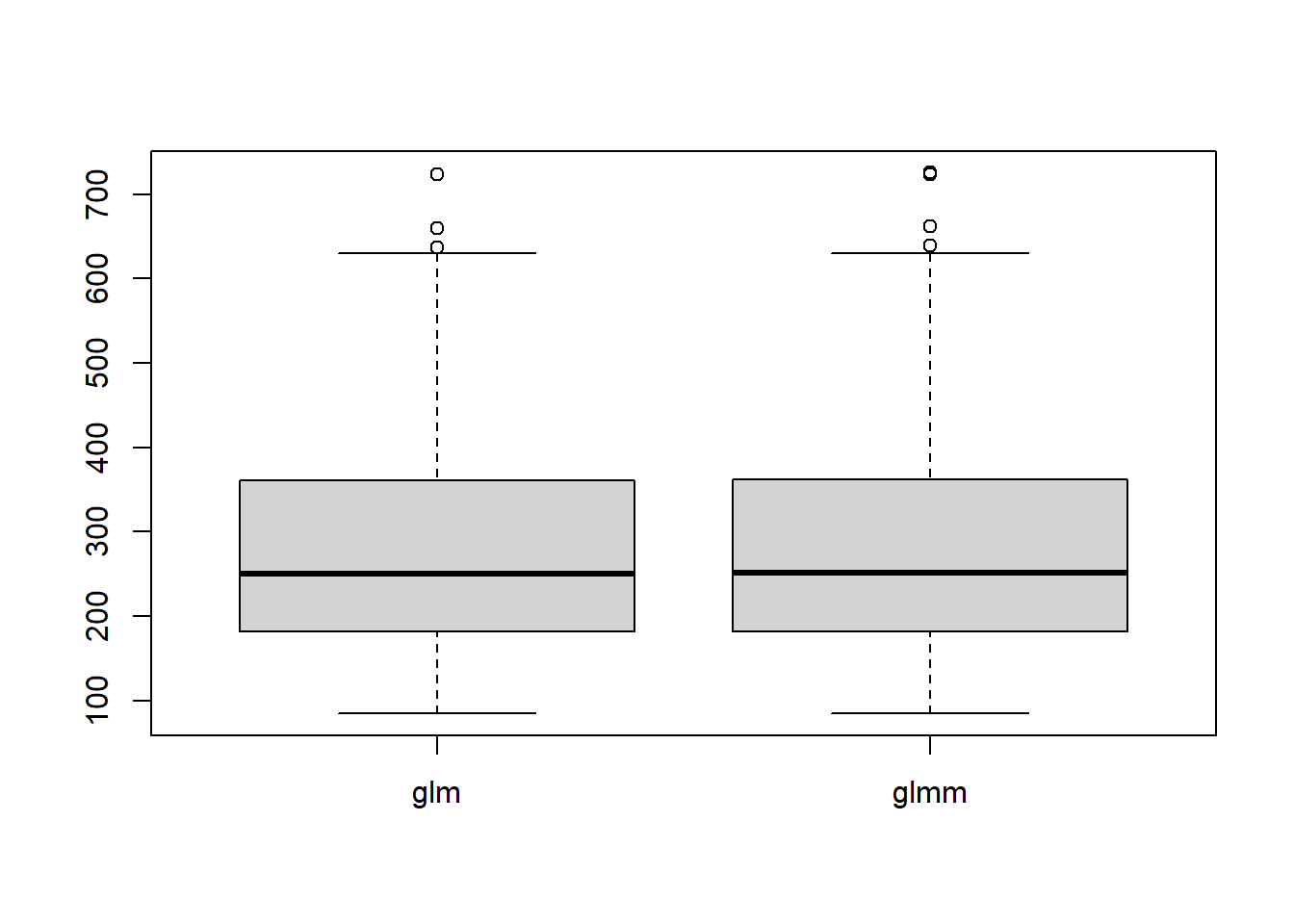In this example the Mixed Model does not improve the prediction quality of the model. This is not surprising since “Zones” is distributed equally and the test dataset resemble the training data very well. The take away message here is that in this example we are not worse off using a glmm instead of a glm.

We will now push the luck a bit in favor n the direction of the GLMM by modifying the sample strategy to evaluate the model. An important application of the GLMM if not the entire population of a factor is observed,

Since we still want to compare both models and a GLM is not able to perform a prediction using an unknown factor not used for training we will give the glm only very few observations for a certain group instead. To evaluate the model, then only observations from that group are used.

trails = 500
n_train = c( rep(2,trails), rep(5,trails),  rep(20,trails),  rep(30,trails) )

runs_z6 <- foreach(n_train=n_train, .combine='cbind' ) %dopar% {
library('lme4')
train_o6=SwedishMotorInsurance[SwedishMotorInsurance["Zone"]!=6,]
data_m6=SwedishMotorInsurance[SwedishMotorInsurance["Zone"]==6,]
sample=sample(1:dim(data_m6), n_train, replace = FALSE, prob = NULL)
train=rbind(train_o6, data_m6[sample,])
test=data_m6[-sample,]
c(n_train/dim(data_m6), model_eval(train, test))
}

data=t(runs_z6)
boxplot( c(data[,2],data[,3]) ~ paste( rep(round(data[,1],2) ,2), c( rep("GLM", length(n_train)), rep("GLMM", length(n_train))) ) , outline=FALSE,ylab="MSE", xlab="Model",cex.axis = 0.6)
rect(0.5,-3,2.5,3300,col="grey90",lty=0)
rect(4.5,-3,6.5,3300,col="grey90",lty=0)
boxplot( c(data[,2],data[,3]) ~ paste( rep(round(data[,1],2) ,2), c( rep("GLM", length(n_train)), rep("GLMM", length(n_train))) ) , outline=FALSE,ylab="MSE", xlab="Model",add=TRUE,cex.axis = 0.6)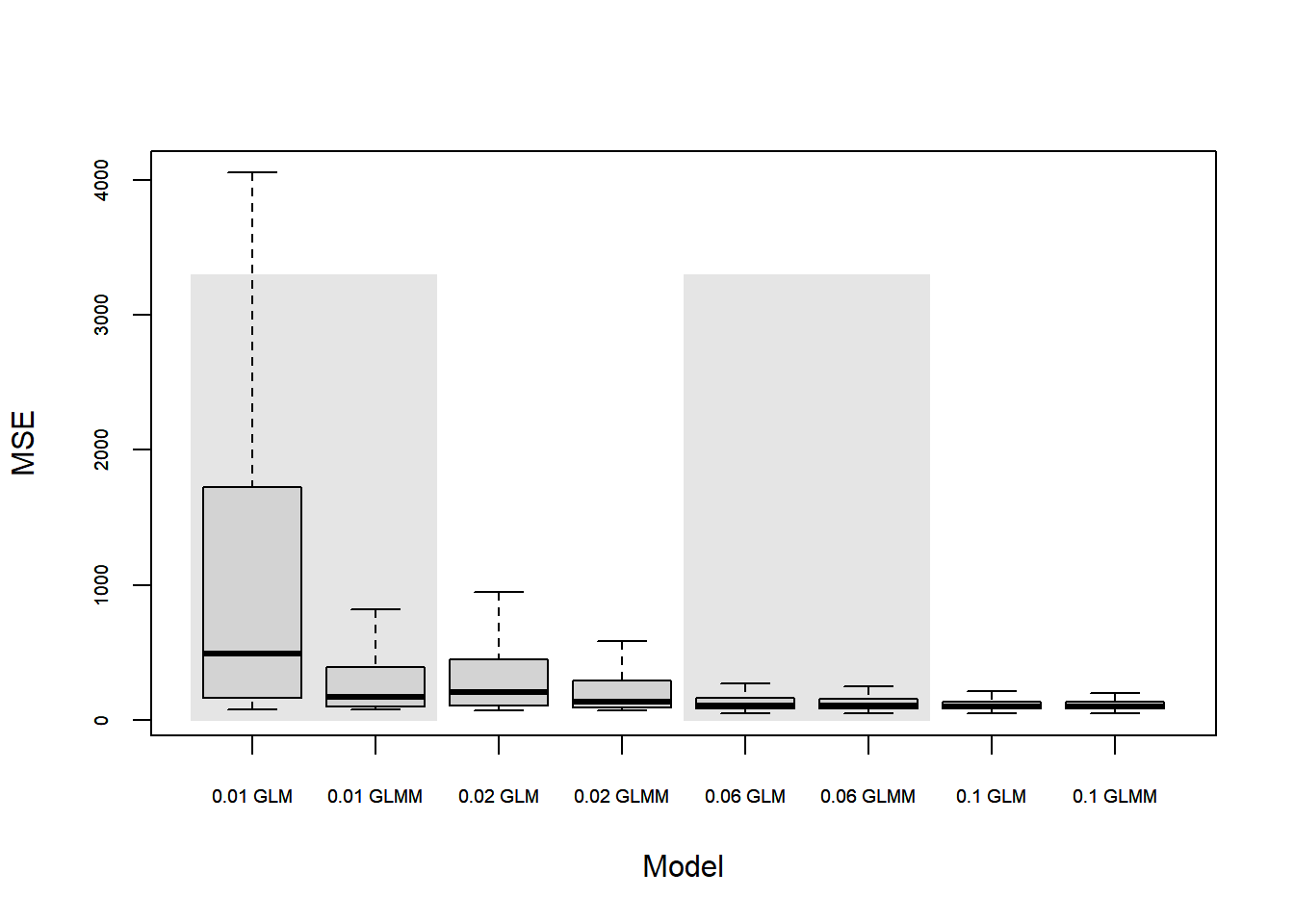The figure shows how the two models perform. For training only a fraction, ranging from 0.01 to 0.1, of observations from one particular group where available. We can conclude, that the GLMM performs better if only a fraction of observation for a certain group are present for training. If more data becomes available the predictive power of GLM and GLMM coincide.

### ReferencesJ. Garrido, C. Genest, and J. Schulz, “Generalized linear models for dependent frequency and severity of insurance claims,” Insurance: mathematics and economics, vol. 70, pp. 205-215, 2016.
[Bibtex]
@article{Garrido2016,
title = {Generalized linear models for dependent frequency and severity of insurance claims},
journal = {Insurance: Mathematics and Economics},
volume = {70},
pages = {205-215},
year = {2016},
issn = {0167-6687},
doi = {https://doi.org/10.1016/j.insmatheco.2016.06.006},
url = {https://www.sciencedirect.com/science/article/pii/S0167668715303358},
author = {J. Garrido and C. Genest and J. Schulz},
keywords = {Aggregate claims model, Claim frequency, Claim severity, Dependence, Exponential dispersion models, Generalized linear model, Loss cost},
abstract = {Traditionally, claim counts and amounts are assumed to be independent in non-life insurance. This paper explores how this often unwarranted assumption can be relaxed in a simple way while incorporating rating factors into the model. The approach consists of fitting generalized linear models to the marginal frequency and the conditional severity components of the total claim cost; dependence between them is induced by treating the number of claims as a covariate in the model for the average claim size. In addition to being easy to implement, this modeling strategy has the advantage that when Poisson counts are assumed together with a log-link for the conditional severity model, the resulting pure premium is the product of a marginal mean frequency, a modified marginal mean severity, and an easily interpreted correction term that reflects the dependence. The approach is illustrated through simulations and applied to a Canadian automobile insurance dataset.}
}S. Haberman and A. E. Renshaw, “Generalized linear models and actuarial science,” Journal of the royal statistical society: series d (the statistician), vol. 45, iss. 4, pp. 407-436, 1996.
[Bibtex]
@article{Haberman1996,
author = {Haberman, Steven and Renshaw, Arthur E.},
title = {Generalized Linear Models and Actuarial Science},
journal = {Journal of the Royal Statistical Society: Series D (The Statistician)},
volume = {45},
number = {4},
pages = {407-436},
keywords = {Generalized linear models, Life-insurance, non-life-insurance models},
doi = {https://doi.org/10.2307/2988543},
abstract = {SUMMARY The authors review the applications of generalized linear models to actuarial problems. This rich class of statistical model has been successfully applied in recent years to a wide range of problems, involving mortality, multiple-state models, lapses, premium rating and reserving. Selective examples of these applications are presented.},
year = {1996}
}
 C. Gao, Q. Li, and Z. Guo, “Automobile insurance pricing with bayesian general linear model,” in Innovative computing and information, Berlin, Heidelberg, 2011, p. 359–365.
[Bibtex]
@InProceedings{Gao2011,
author="Gao, Cheng
and Li, Qi
and Guo, Zirui",
editor="Dai, Minli",
title="Automobile Insurance Pricing with Bayesian General Linear Model",
booktitle="Innovative Computing and Information",
year="2011",
publisher="Springer Berlin Heidelberg",
pages="359--365",
abstract="This paper applies Bayesian Model to the automobile insurance pricing in the purpose of solving the problem that the Generalized Linear Model (GLM) applied in actuarial pricing cannot reveal prior information and has too much confidence in the information from data. Under the assumption of Squared Error Loss, the estimation of the parameter in the model is the mean of the posterior distribution which was calculated by Markov Chain Monte Carlo Methods (MCMC). Finally, take the auto-insurance of WASA Insurance Company in Switzerland as an example. Run MCMC in WINBUGS software to get the estimation of the parameters, and design the comparative auto insurance tariff for this company. The comparison between the pricing utilizing Bayesian Model and that according to GLM reveals that owing to the function of prior information, the two automobile tariffs differ distinctively. Moreover, the prior information has been elegantly reflected in the Bayesian Model.",
isbn="978-3-642-23993-9"
}L. Guelman, “Gradient boosting trees for auto insurance loss cost modeling and prediction,” Expert systems with applications, vol. 39, iss. 3, pp. 3659-3667, 2012.
[Bibtex]
@article{Guelman2012,
title = {Gradient boosting trees for auto insurance loss cost modeling and prediction},
journal = {Expert Systems with Applications},
volume = {39},
number = {3},
pages = {3659-3667},
year = {2012},
issn = {0957-4174},
doi = {https://doi.org/10.1016/j.eswa.2011.09.058},
url = {https://www.sciencedirect.com/science/article/pii/S0957417411013674},
author = {Leo Guelman},
keywords = {Statistical learning, Gradient boosting trees, Insurance pricing},
abstract = {Gradient Boosting (GB) is an iterative algorithm that combines simple parameterized functions with “poor” performance (high prediction error) to produce a highly accurate prediction rule. In contrast to other statistical learning methods usually providing comparable accuracy (e.g., neural networks and support vector machines), GB gives interpretable results, while requiring little data preprocessing and tuning of the parameters. The method is highly robust to less than clean data and can be applied to classification or regression problems from a variety of response distributions (Gaussian, Bernoulli, Poisson, and Laplace). Complex interactions are modeled simply, missing values in the predictors are managed almost without loss of information, and feature selection is performed as an integral part of the procedure. These properties make GB a good candidate for insurance loss cost modeling. However, to the best of our knowledge, the application of this method to insurance pricing has not been fully documented to date. This paper presents the theory of GB and its application to the problem of predicting auto “at-fault” accident loss cost using data from a major Canadian insurer. The predictive accuracy of the model is compared against the conventional Generalized Linear Model (GLM) approach.}
}R. Henckaerts, M. Côté, K. Antonio, and R. Verbelen, “Boosting insights in insurance tariff plans with tree-based machine learning methods,” North american actuarial journal, pp. 1-31, 2020.
[Bibtex]
@article{Henckaerts2020,
author = { Roel Henckaerts and Marie-Pier Côté and Katrien Antonio and Roel Verbelen },
title = {Boosting Insights in Insurance Tariff Plans with Tree-Based Machine Learning Methods},
journal = {North American Actuarial Journal},
volume = {0},
number = {0},
pages = {1-31},
year = {2020},
publisher = {Routledge},
doi = {10.1080/10920277.2020.1745656},
URL = {
https://doi.org/10.1080/10920277.2020.1745656
},
eprint = {
https://doi.org/10.1080/10920277.2020.1745656
}
}

This site uses Akismet to reduce spam. Learn how your comment data is processed.